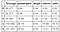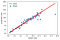# 1. Introduction

Using the cruise ship dataset cruise_ship_info.csv, we will demonstrate simple and multiple regression analysis using NumPy, Pylab, and Scikit-learn. Because this is just an introductory tutorial, no distinction between inliers and outliers shall be made (outliers can be handled using more robust methods such as the RANSAC regression).

# 2. Data Analysis

## 2.1 Import Necessary Libraries

`import numpy as npimport pandas as pdimport pylabimport matplotlib.pyplot as pltimport seaborn as snsimport matplotlib.pyplot as pltfrom sklearn.metrics import r2_scorefrom sklearn.model_selection import train_test_splitfrom sklearn.preprocessing import StandardScalerfrom sklearn.linear_model import LinearRegressionfrom sklearn.pipeline import Pipelinepipe_lr = Pipeline([('scl', StandardScaler()),                    ('lr', LinearRegression())])`

## 2.2 Read dataset and display columns

`df = pd.read_csv("cruise_ship_info.csv")df.head()`

## 2.3 Calculate the covariance matrix

`cols = ['Age', 'Tonnage', 'passengers', 'length',                       'cabins','passenger_density','crew']stdsc = StandardScaler()X_std = stdsc.fit_transform(df[cols].iloc[:,range(0,7)].valuescov_mat = np.cov(X_std.T)`

## 2.4 Generate a heatmap for visualizing the covariance matrix

`plt.figure(figsize=(10,10))sns.set(font_scale=1.5)hm = sns.heatmap(cov_mat,                 cbar=True,                 annot=True,                 square=True,                 fmt='.2f',                 annot_kws={'size': 12},                 yticklabels=cols,                 xticklabels=cols)plt.title('Covariance matrix showing correlation coefficients')plt.tight_layout()plt.show()`

# 3. Simple Linear Regression

where m is the slope or regression coefficient, and c is the intercept. The model will be evaluated using the R2 score metric which can be calculated as follows:

The R2 score takes values between 0 and 1. When R2 is close to 1, it means the predicted values agree closely with the actual values. If R2 is close to zero, then it means the predictive power of the model is very poor.

Let’s now define and plot our independent and dependent variables:

`X = df['cabins']y = df['crew']plt.scatter(X,y,c='steelblue', edgecolor='white', s=70)plt.xlabel('cabins')plt.ylabel('crew')plt.title('scatter plot of crew vs. cabins')plt.show()`

## 3.1 Simple linear regression using numpy

`z = np.polyfit(X,y,1)p = np.poly1d(z)print(p)`

Output: 0.745 x + 1.216

This shows that the fitted slope is m = 0.745, and the intercept is c = 1.216.

`y_pred_numpy = p(X)R2_numpy = 1 - ((y-y_pred_numpy)**2).sum()/((y-y.mean())**2).sum()print(R2_numpy)`

Output: R2_numpy = 0.9040636287611352

`print(r2_score(y, y_pred_numpy))`

Output: 0.9040636287611352

Let’s now plot the actual and predicted values:

`plt.figure(figsize=(10,7))plt.scatter(X,y,c='steelblue', edgecolor='white', s=70,              label='actual')plt.plot(X,y_pred_numpy, color='black', lw=2, label='predicted')plt.xlabel('cabins')plt.ylabel('crew')plt.title('actual and fitted plots')plt.legend()plt.show()`

## 3.2 Simple linear regression using Pylab

`degree = 1model= pylab.polyfit(X,y,degree)print(model)`

Output: array([0.7449974 , 1.21585013]). We see again that the slope is m = 0.745, and the intercept is c = 1.216.

`y_pred_pylab = pylab.polyval(model,X)R2_pylab = 1 - ((y-y_pred_pylab)**2).sum()/((y-y.mean())**2).sum()print(R2_pylab)`

Output: R2_pylab = 0.9040636287611352

`print(r2_score(y, y_pred_pylab))`

Output: 0.9040636287611352

## 3.3 Simple linear regression using scikit-learn

`lr = LinearRegression()lr.fit(X.values.reshape(-1,1),y)print(lr.coef_)print(lr.intercept_)`

Output: [0.7449974], 1.2158501299368671. We see again that the slope is m = 0.745, and the intercept is c = 1.216.

`y_pred_sklearn = lr.predict(X.values.reshape(-1,1))R2_sklearn = 1 - ((y-y_pred_sklearn)**2).sum()/((y-y.mean())**2).sum()print(R2_sklearn)`

Output: R2_sklearn = 0.9040636287611352

`print(r2_score(y, y_pred_sklearn))`

Output: 0.9040636287611352

We observe that all 3 methods for basic linear regression (NumPy, Pylab, and Scikit-learn) gave consistent results.

# 4. Multiple Linear Regression with Scikit-Learn

where X is the features matrix, w_0 is the intercept, and w_1, w_2, w_3, and w_4 are the regression coefficients.

## 4.1 Define features matrix and the target variable

`cols_selected = ['Tonnage', 'passengers', 'length', 'cabins','crew']df[cols_selected].head()X = df[cols_selected].iloc[:,0:4].values    # features matrix y = df[cols_selected]['crew'].values        # target variable`Table 2. First 5 rows of important features and predictor variables.

## 4.2 Model building and evaluation

`X_train, X_test, y_train, y_test = train_test_split( X, y, test_size=0.3, random_state=0)sc_y = StandardScaler()y_train_std = sc_y.fit_transform(y_train[:,np.newaxis]).flatten()pipe_lr.fit(X_train, y_train_std)y_train_pred = sc_y.inverse_transform(pipe_lr.predict(X_train))y_test_pred = sc_y.inverse_transform(pipe_lr.predict(X_test))r2_score_train = r2_score(y_train, y_train_pred)r2_score_test = r2_score(y_test, y_test_pred)print('R2 train for lr: %.3f' % r2_score_train)print('R2 test for lr:  %.3f ' % r2_score_test)`

Output:

R2 train for lr: 0.912
R2 test for lr: 0.958

## 4.3 Plot the output

`plt.scatter(y_train, y_train_pred, c='steelblue', edgecolor='white', s=70, label='fitted')plt.plot(y_train, y_train, c = 'red', lw = 2,label='ideal')plt.xlabel('actual crew')plt.ylabel('predicted crew')plt.legend()plt.show()`Figure 4. Ideal and fitted plots for the crew variable using multiple regression analysis.

# Additional Data Science/Machine Learning Resources

Data Science Curriculum

Essential Maths Skills for Machine Learning

3 Best Data Science MOOC Specializations

5 Best Degrees for Getting into Data Science

5 reasons why you should begin your data science journey in 2020

Theoretical Foundations of Data Science — Should I Care or Simply Focus on Hands-on Skills?

Machine Learning Project Planning

How to Organize Your Data Science Project

Productivity Tools for Large-scale Data Science Projects

A Data Science Portfolio is More Valuable than a Resume

Data Science 101 — A Short Course on Medium Platform with R and Python Code Included

For questions and inquiries, please email me: benjaminobi@gmail.com

Written by

Written by

## Benjamin Obi Tayo Ph.D.

#### Physicist, Data Science Educator, Writer. Interests: Data Science, Machine Learning, AI, Python & R, Predictive Analytics, Materials Sciences, Biophysics